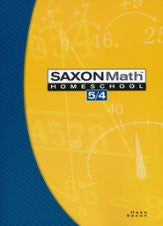# Math 5/4 Homeschool Student Edition (3rd Edition)

• \$ 56.80

Covers concepts such as whole number concepts and computation; arithmetic algorithms, geometry and measurement; elapsed time; fractions, decimals and percents; powers and roots; estimation; patterns and sequences; congruency and similarity; and statistics and probability. Students will specifically learn to add three-digit numbers, subtract numbers with re-grouping, read time, write numbers, estimate arithmetic answers, divide with two-digit answers, multiply three or more factors, simplify fraction answers, use a decimal number line, and more.

Lessons contain a warm-up (with facts practice, mental math, & problem-solving exercises), an introduction to the new concept, lesson practice exercises where the new skill is practiced, and mixed practice exercises, which include 25-30 old and new problems. In-depth "Investigations" are provided every 10 lessons, and have their own set of questions. Math 5/4 also includes 8 in-lesson activities and 5 investigation activities. The back of the book contains supplemental problems for selected lessons and concepts.

627 pages, softcover. Consumable workbook. Reproduction of most pages is prohibited.